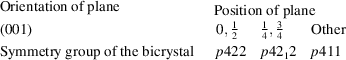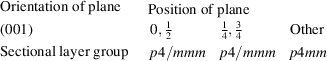International
Tables for
Crystallography
Volume E
Subperiodic groups
Edited by V. Kopský and D. B. Litvin

International Tables for Crystallography (2010). Vol. E, ch. 5.2, pp. 414-415   | 1 | 2 |

Section 5.2.5.2. Interfaces in crystalline materials

V. Kopskýa* and D. B. Litvinb

aFreelance research scientist, Bajkalská 1170/28, 100 00 Prague 10, Czech Republic, and bDepartment of Physics, The Eberly College of Science, Penn State – Berks Campus, The Pennsylvania State University, PO Box 7009, Reading, PA 19610–6009, USA
Correspondence e-mail:  kopsky@fzu.cz

5.2.5.2. Interfaces in crystalline materials

| top | pdf |

The scanning for the sectional layer groups is a procedure which finds applications in the theory of bicrystals and their interfaces. The first of these two terms was introduced in the study of grain boundaries (Pond & Bollmann, 1979; Pond & Vlachavas, 1983; Vlachavas, 1985; Kalonji, 1985). An ideal bicrystal is understood to be an aggregate of two semi-infinite crystals of identical structure, meeting at a common planar boundary called the interface, where one of the structures, occupying half-space on one side of the interface, is misoriented and/or displaced relative to the other structure occupying the other half-space. The word interface is a synonym for a boundary and interfaces considered here are homophase interfaces, in contrast with heterophase interfaces, where the two structures are different (Sutton & Balluffi, 1995).

An independent study of domain and twin boundaries (Janovec, 1981; Zikmund, 1984) resulted in a terminology parallel to that of the bicrystallography. The basic concept here is the domain twin, which is technically a particular case of a bicrystal. In this section, we use the terminology of bicrystals, giving the terminology of domain twins, used in the next section, parenthetically.

In both cases, the aim of the analysis is to determine the symmetry group of a bicrystal (domain twin), corresponding to a certain orientation and location of the interface (domain wall or twin boundary), which is a certain layer group.

The bicrystal (domain twin) is a conceivable real structure in space!

In the first step of the analysis, one constructs a dichromatic complex or pattern [(unordered) domain pair].

The dichromatic complex (domain pair) is not a real structure!

It is an abstract construction, a superposition of two infinite crystals which have the same structure, orientation and/or location as the two semi-infinite crystals of the bicrystal (domain twin) when extended to infinity. The two components are referred to as black and white crystals or variants (single domain states).

The symmetry groupof the dichromatic complex (domain pair) is the group of those Euclidean motions which either leave both black and white crystals (domain states) invariant or which exchange them. Planes of various orientations and locations, representing the interface, are then considered as transecting the dichromatic complex (domain pair). To each such plane there corresponds a sectional layer group, the elements of which leave invariant the dichromatic pattern (domain pair) and the plane. A bicrystal (domain twin) is obtained by deleting from one side of the plane the atoms of one of the components of the dichromatic pattern (single domain states) and the atoms of the second component (single domain state) from the other side of the plane. The symmetry of the bicrystal (domain twin) is a layer group which contains those elements of the sectional layer group of the dichromatic pattern (domain pair) that satisfy one of the following two conditions:

 (i) elements that leave invariant both the black and white crystals (both single domain states) and the normal to the plane; (ii) elements that exchange the black and white crystals (single domain states) and invert the normal to the plane.

Example

Consider the bicrystal consisting of two face-centred cubic crystals misoriented by a rotation of 36.9° about the  direction. The corresponding dichromatic complex is shown in Fig. 5.2.5.3. The symmetry group of the complex is the space group,(No. 139).Figure 5.2.5.3 | top | pdf |A classical example of a bicrystal (Vlachavas, 1985).

Vlachavas (1985) has tabulated the symmetries of bicrystals arising when the above dichromatic complex is transected with planes of various orientations and locations. For planes of the orientation (001), given with reference to the tetragonal coordinate system shown in Fig. 5.2.5.3, Vlachavas listsThe position of the plane is given in terms of a fraction of the basis vector of the tetragonal c axis. The p' in the symbol of the symmetry groups of the bicrystal denotes all translations in the (001) plane.

From the subtable for the space group,(No. 139) in the scanning tables, Part 6, one findsThe symmetry group of the bicrystal is that subgroup of the corresponding sectional layer group consisting of all elements that satisfy one of the two conditions given above. For example, for the plane at position 0', the sectional layer group is(L61). None of the mirror planes satisfies either of the conditions. The mirror plane perpendicular to  inverts the normal to the plane but leaves invariant both black and white crystals. The mirror planes perpendicular to  and  leave the normal to the plane invariant, but exchange the black and white crystals. The fourfold rotation satisfies condition (i), and the twofold rotations about auxiliary axes satisfy condition (ii). Consequently, from the sectional layer groups(L61),(L64) and(L55) one obtains the respective symmetries of the bicrystal with different locations of interfaces:(L53),(L54) and(L49), as listed by Vlachavas.

References

Janovec, V. (1981). Symmetry and structure of domain walls. Ferroelectrics, 35, 105–110.
Kalonji, G. (1985). A roadmap for the use of interfacial symmetry groups. J. Phys. C, 46, 249–256.
Pond, R. C. & Bollmann, W. (1979). The symmetry and interfacial structure of bicrystals. Philos. Trans. R. Soc. London Ser. A, 292, 449–472.
Pond, R. C. & Vlachavas, D. S. (1983). Bicrystallography. Proc. R. Soc. London Ser. A, 386, 95–143.
Sutton, A. P. & Balluffi, R. W. (1995). Interfaces in crystalline materials. Oxford: Clarendon Press.
Vlachavas, D. S. (1985). Symmetry of bicrystals corresponding to a given misorientation relationship. Acta Cryst. A41, 371–376.
Zikmund, Z. (1984). Symmetry of domain pairs and domain twins. Czech. J. Phys. B, 34, 932–949.